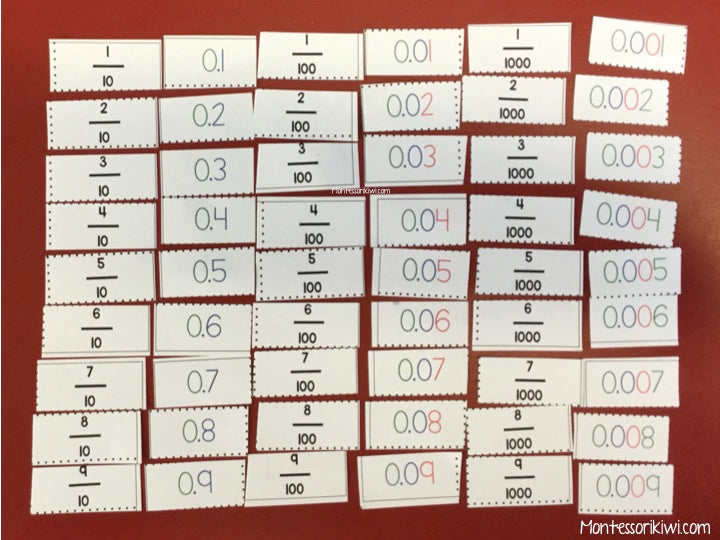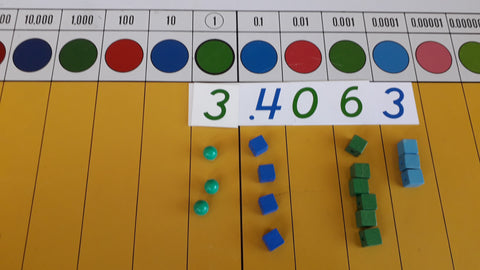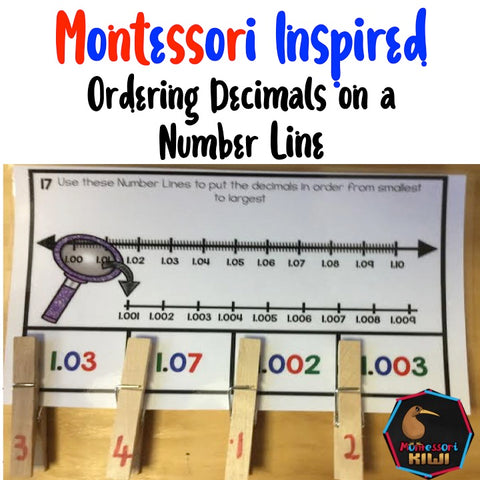# Introduction to the Montessori Decimal Board Part 3

Earlier I talked a bit about how we can introduce the Montessori decimal board here (part 1) and  here (part 2)

In the last section of Part 2 the lesson was on the student exchanging  say 12 tenths for one whole and two tenths to make 1.2.

The Lesson: Making larger numbers on the decimal board

The next step is to ask the student to make bigger amounts on the decimal board

You can do this a number of ways for example the below amount could be made by asking the student to do one of the following

• Make three wholes plus four tenths plus six thousandths plus three ten thousandths
• Make 3 + 4/10 + 6/1000 + 3/10 000
• Make the number that is one whole more than 2.4063
• Make the number that is one tenth more than 3.3063The Lesson: Rounding numbers

Dependent on where you are students may be familiar with rounding due to the currency you use for example \$3.46. You could ask them whether 3.4 is closer to 3 or closer to 4. Having a number line to illustrate is really helpful along with making the number on the decimal board. When students are becoming more confident this activity is helpfulNow is a good time to give students lots of opportunity to practise everything they have learnt so far.  These task cards are ideal and help students:

Convert simple fractions to decimals
Know how many tenths in a number
Convert simple percentages to decimals
Know how many tenths are in a number
Round decimals
Know if a decimal is closer to one number or another
Know whether a certain fraction or decimal is smaller or larger
Know how many hundredths are in a number
Find a decimal number halfway between two numbers

The Lesson:  Showing how decimals and fractions look in different notations

This is also a good time to reinforce how decimals relate to fractions by asking students to write or demonstrate a fraction or decimal in the other notation. This match up activity worked well in my class.In addition having students order numbers on a numberline with decimals and fractions is really helpful. Here I made a giant numberline that went from nearly one side of the class to the other from 0.0 - 1.0. I marked it at every tenth. I then gave out a range of premade cards and students placed them on the numberline. We then turned the cards over and wrote the fractional number.For the earlier parts in this series on the decimal board